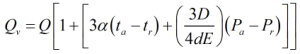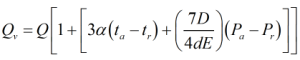# C170 – Instromet – Ultrasonic Meter Flowrate

## Description

This calculates the volume flow rate corrected for the temperature and pressure of the spool piece when using an Instromet ultrasonic meter.

Temperature and pressure correction for ultrasonic gas flow meters, Geeuwke de Boer & Jan G Drenthen: Instromet International N.V.

Kelton calculation reference C170

FLOCALC Calculation reference F039

KIMS Calculation reference K176

Kelton calculation C244 – Calibration Correction

## Options

### Spool Correction Factor

• User entered

Select this option to enter a single multiplier for the correction factor – if no correction is made set this value to 1

• Calculated

Select this option to calculate the correction factors using the manufacturers equations

### Calibration Correction

• None

Select this option if you do not wish to correct the flow rate for the calibration curve

• Before Spool Correction

Select this option if you wish to correct the flow rate based on the calibration data before applying the correction for spool temperature and pressure

• After Spool Correction

Select this option if you wish to correct the flow rate based on the calibration data after applying the correction for spool temperature and pressure

The correction is carried out as per C244.

### Spool Piece

• Flanged

Select this option for flanged spool piece meters

• Welded

Select this option for welded spool piece meters

### Calculate for

The calculated flow rate can be expressed in:

• Observed volume flow rate
• Mass flow rate
• Standard volume flow rate
• Normal volume flow rate
• Energy flow rate

## Calculations

All calculations are performed in SI Units unless otherwise stated

If the flanged spool piece option is chosenIf the welded spool piece option is chosenWhere Q = The displayed volume flow rate α = The temperature coefficient for the spool ta = The spool temperature tr = The reference spool temperature D = The internal meter diameter d = The meter wall thickness E = Young’s modulus of elasticity Pa = The spool pressure Pr = The reference spool pressure

Back